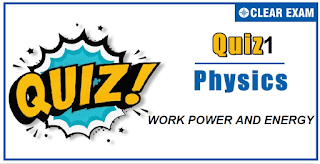## [LATEST]\$type=sticky\$show=home\$rm=0\$va=0\$count=4\$va=0

As per analysis for previous years, it has been observed that students preparing for NEET find Physics out of all the sections to be complex to handle and the majority of them are not able to comprehend the reason behind it. This problem arises especially because these aspirants appearing for the examination are more inclined to have a keen interest in Biology due to their medical background.

Furthermore, sections such as Physics are dominantly based on theories, laws, numerical in comparison to a section of Biology which is more of fact-based, life sciences, and includes substantial explanations. By using the table given below, you easily and directly access to the topics and respective links of MCQs. Moreover, to make learning smooth and efficient, all the questions come with their supportive solutions to make utilization of time even more productive. Students will be covered for all their studies as the topics are available from basics to even the most advanced.

Q1. The decrease in the potential energy of a ball of mass 20 kg which falls from a height of 50 cm is
•  968J
•  98J
•  1980J
•  None of them
Solution
(b) ∆U=mgh=20×9.8×0.5=98 J

Q2.The potential energy of a certain spring when stretched through a distance ‘S’ is 10 joule. The amount of work (in joule) that must be done on this spring to stretch it through an additional distance ‘S’ will be
•  30
•  40
•  10
•  20
Solution
(a) 1/2 kS2=10 J [Given in the problem] 1/2 k[(2S)2-(S)2]=3×1/2 kS2=3×10=30 J

Q3.  One man takes 1 minute to raise a box to a height of 1 m and another man takes 1/2 minute to do so. The energy of the two is
•  Different
•  same
•  Energy of First is more
•  Energy of second is more
Solution
(b) Energy required =mgh In both cases, h is the same. Hence, energy given by both is same. [It is worth noting here that powers of two men will be different as power is the energy expense per unit time and times are different]

Q4. The potential energy function for the force between two atoms in a diatomic molecule is approximately given by U(x)=a/x12 -b/x6 ,where a and b are constants and x is the distance between the atoms. If the dissociation energy of the molecule is D=[U(x=∞)-U(at equilibrium)], D is
•  b22/6a
•  b22/2a
•  b22/12a
•  b22/4a
Solution
U= a/x12 -b/x6 F=-dU/dx=+12a/x13-6b/x7 =0⇒x=(2a/b)1/6 U(x=∞)=0 Uequilibrium=a/(2a/b)2-b/((2a/b) )=b2/4a ∴U(x=∞)-Uequilibrium=0-(-b2/4a)=b2/4a

Q5.If the kinetic energy of a body is increased 2 times , its momentum will
•  Half
•  Remain Unchanged
•  Be doubled
•  increase√2 times
Solution
Kinetic energy of particle ,k=(p12/2m p12=2mk^' When kinetic energy =2k p22=2m×2k,p22=2p12,p2=√(2p_1 )

Q6. A steel ball of radius 2 cm is at rest on a frictionless surface. Another ball of radius 4 cm moving at a velocity of 81 cm/sec collides elastically with first ball. After collision the smaller ball moves with speed of
•  81cm/sec
•  63 cm/sec
• 144 cm/sec
•  None of These
Solution
Radio in radius of steel balls =1/2 So, ratio in the masses =1/8 [As M∝V∝r3] Let m1=8m and m2=m
v2=(2m1u1)/(m1+m2)=(2×8m×81)/(8m+m)=144 cm/s

Q7.If W1,W2 and W3represent the work done in moving a particle from A to B along three different paths 1, 2 and 3 respectively (as shown) in the gravitational field of a point mass m, find the correct relation between W1,W2 and W3

•  W1>W2>W3
•  W1=W2=W3
•  W1< W2< W3
•  W2>W1>W3
Solution
(b) Gravitational force is a conservative force and work done against it is a point function i.e. does not depend on the path

Q8.Two putty balls of equal mass moving with equal velocity in mutually perpendicular directions, stick together after collision. If the balls were initially moving with a velocity of 45√2 ms-1each, the velocity of their combined after collision is
•  45√2 ms-1
•  T45ms-1
•  90ms-1
•  22.5√2 ms-1
Solution

P->=m45√2 i ̂+m45√2 j^ ⇒|P ⃗ |=m×90 Final momentum 2m×V By conservation of momentum 2m×V=m×90 ∴V=45 m/s

Q9.The force constant of a wire is k and that of another wire is 2k. When both the wires are stretched through same distance, then the work done
•  W2=2 W12
•  W2=2W1
•  W2=W1
•  W2=0.5 W1
Solution
(b) W=1/2 kx2 If both wires are stretched through same distance then W∝k. As k2=2k1 so W2=2W1

Q10. A man does a given amount of work in 10 s. Another man does the same amount of work in 20 s. The ratio of the output power of first man to the second man is
•  1
•  1/2
•  2/1
• None of these
Solution
(c) Given ,t1=10s, t2=20, w1=w2 power=(work done)/time or p1/p2 =(w1/t1)/(w2/t2) ∴ p1/p2 =t2/t1 =2/1#### Written by: AUTHORNAME

AUTHORDESCRIPTION## Want to know more

Please fill in the details below:

## Latest NEET Articles\$type=three\$c=3\$author=hide\$comment=hide\$rm=hide\$date=hide\$snippet=hide

Name

ltr
item
BEST NEET COACHING CENTER | BEST IIT JEE COACHING INSTITUTE | BEST NEET, IIT JEE COACHING INSTITUTE: Work-Power-And-Energy-Quiz-1
Work-Power-And-Energy-Quiz-1